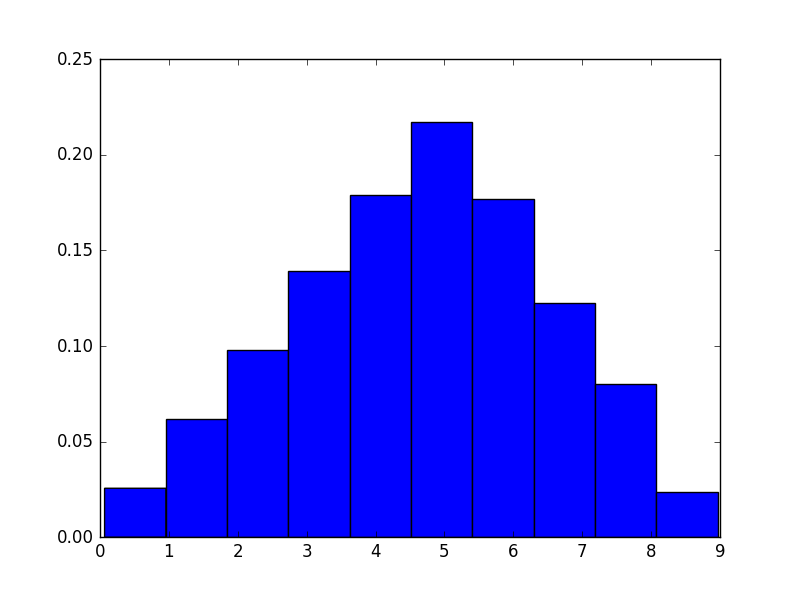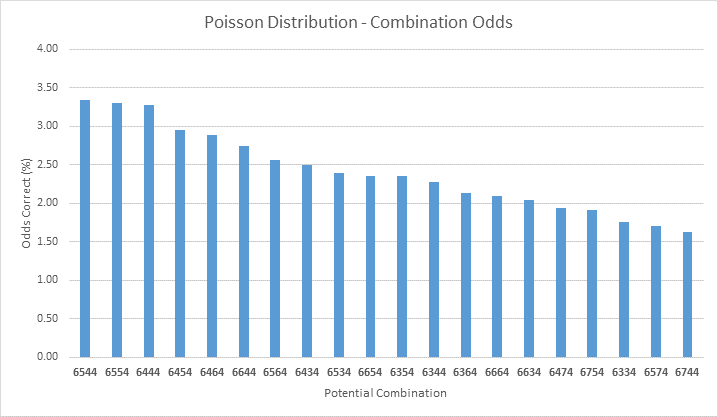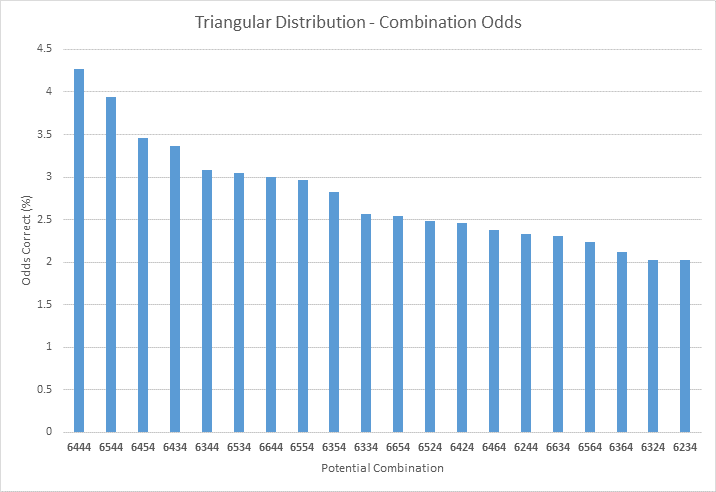# Background

My dad recently came to me with an interesting proposition - cracking a combination lock where the first and last numbers are (probably) known. He has a lock where the first and last digits are 6 and 4, respectively, but is looking to determine the middle two digits and hopefully crack the overall combination.

My first thought was to simply generate all the possibilities of numbers - a relatively trivial task, easy but long. This, however, wouldn’t have any sort of predictive aspect at all - when randomly generated, the probability of a 6004 would be the same as a 6474.

In search of a better model, I turned to the Poisson and Triangular number distributions. The shape of these distributions varies, but each relates to a Probability Distribution Function (PDF) which is weighted around a target (we’ll call it a seed) value. I figured that if we could supply a decent guess for the seed value, we’d be able to increase the probability of our combinations being correct, and minimize the probability of choosing a combination drastically different from the seed value.

# Approach

Ordinary combination locks have four dials, where each number is a value between 0 and 9. This reveals a few properties:

• Potential combinations: 104
• Mean sum of digits: 18

As I mentioned earlier, my Dad was pretty confident that the first and last values of the combination were 6 and 4, meaning our potential combinations are going to be in the format of 6##4. It made sense to me that the remaining digits would be relatively close to 6 and 4, in an effort to minimize dial traffic/rotation for whoever is inputting the combinations. With this in mind, I chose 5 as the seed value for each of the digits - guessing that this would be the best place to center our distributions and making the implicit inference that the lock-setter would rather pick a 5 than making the additional turns for a 1 or a 9.

``````# Import statements
import numpy as np
import matplotlib.pyplot as plt
from collections import Counter

# Numbers found: 6164, 6474
COMBO_FIRST = 6
COMBO_FOURTH = 4

# Guess at the seed value
SEED = 5
``````

# Execution

To generate the combinations, I chose to draw my numbers from the Poisson and Triangular distributions (more details below). For each combination, I used Numpy’s number generators to randomly generate viable second and third digits for the combination from the number distribution of choice, then concatenating those numbers with the first and fourth digits to create a combination “guess”. I repeated this process 10,000 times for each distribution in a Monte Carlo simulation with the goal of determining which lock combinations are the most common and therefore likely to be true in real life.

# Generate Combinations with Poisson Distribution

Per Wikipedia, Poisson distributions are excellent for “modeling the number of times an event occurs in a given interval of time or space”, especially when the average number of events in an interval is known. In our case, assuming that the most likely digit on the lock would be a five, the Poisson distribution will center the distribution of guesses around five.``````# This function generates a four digit lock combination
# using a Poisson function to create random values for the
# second and third lock digits
def genPoissionComb(lam):
num2 = np.random.poisson(lam=lam, size=1)
num3 = np.random.poisson(lam=lam, size=1)
# Remove number possibilities > 9, as Poisson is unbounded
if (num2 > 9 or num3 > 9):
return
combo = str(COMBO_FIRST) + str(num2) +
str(num3) + str(COMBO_FOURTH)
return combo
``````
``````# This function creates a list of feasible lock combinations
# from our Poisson generator
def createPoissonDist(size):
pdist = []
for i in range(1,size):
n = genPoissionComb(seed)
if (n == None):
continue
pdist.append(n)
return pdist
``````

# Generate Combinations with Triangular Distribution

The Triangular Distribution is named for the shape of its PDF - a triangle centered around a mode, with upper and lower bounds. In our case, we can assume the mode to be our seed value (5), and the upper and lower bounds to be the edges of the combination lock (0 and 9).``````# This function generates a four digit lock combination
# using a Triangular function to create random values for the
# second and third lock digits
def genTriangularComb(mode):
num2 = np.random.triangular(0, mode, 9, 1)
num3 = np.random.triangular(0, mode, 9, 1)
combo = str(COMBO_FIRST) + str(int(num2)) +
str(int(num3)) + str(COMBO_FOURTH)
return combo
``````
``````# This function creates a list of feasible lock combinations
# from our Triangular generator
def createTriangularDist(size):
tdist = []
for i in range(1,size):
tdist.append(genTriangularComb(SEED))
return tdist
``````

# Results

After running a 10,000 guess Monte Carlo simulation for each probability distribution, I collected the most common combinations and determined their likelihood. Check out the results below - my dad is going to test the top 20 combinations for each method and see what works!